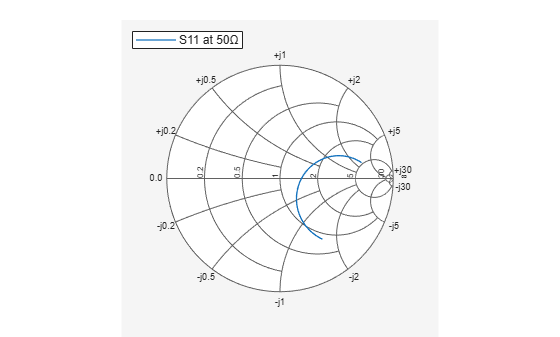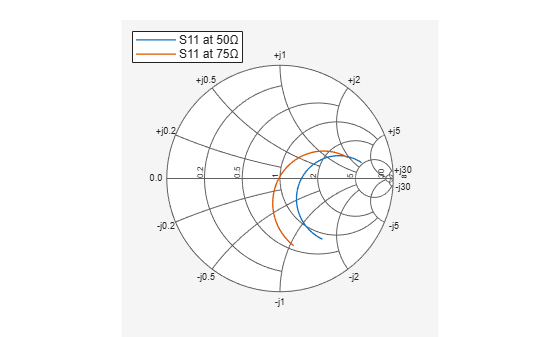## Syntax

``add(plot,data)``
``add(plot,frequency,data)``

## Description

````add(plot,data)` adds data to an existing Smith chart.```
````add(plot,frequency,data)` adds data to an existing Smith chart based on multiple data sets containing frequencies corresponding to columns of data matrix.```

## Examples

collapse all

Plot the reflection coefficients of a dipole antenna.

Create a strip dipole antenna on the Y-Z plane. Calculate the complex s-parameters of the dipole antenna from 60 MHz to 90 MHz, with an interval of 150 kHz.

Plot S11 on a Smith plot for a reference impedance of 50 ohm.

```d = dipole; freq = linspace(60e6, 90e6, 200); s_50 = sparameters(d, freq,50); hg = smithplot(s_50,[1,1]); hg.LegendLabels = {"S11 at 50#ohm"};```Find S11 for a new impedance of 75 ohm. Add new S11 to the existing Smith plot.

```s_75 = sparameters(d, freq, 75); gamma = rfparam(s_75,1,1); add(hg, gamma); hg.LegendLabels = {"S11 at 50#ohm","S11 at 75#ohm"};```## Input Arguments

collapse all

Smith chart handle, specified as a function handle. If the handle of the Smith chart is not retained during creation, it is obtained by using the command `p = smithplot('gco')`.

Data Types: `double`

Input data, specified as a complex vector or complex matrix.

For a matrix D, the columns of D are independent data sets. For N-by-D arrays, dimensions 2 and greater are independent data sets.

Data Types: `double`
Complex Number Support: Yes

Frequency data, specified as a real vector.

Data Types: `double`

## Version History

Introduced in R2017b